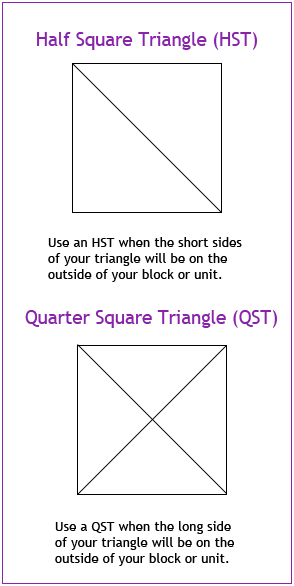## Half Square Triangles (HSTs) and Quarter Square Triangles (QSTs): What’s the difference?

I’ve been asked to repost my explanation of the difference between Half Square Triangles and Quarter Square Triangles. Here it is:Half square and quarter square triangles differ in several key ways. At the most basic level, they differ in the number of times you cut the square diagonally. A half square triangle is cut only once; a quarter square triangle is cut diagonally twice.

How many times you cut the square not only affects the size of the triangle but also determines where the bias edges of the triangle will be. When you cut a square in half, the two sides that form the right angle of the resulting triangle (the short sides) are on the grain line. When you cut a square in quarters the only side on the grain line is the long side opposite the right angle.

Why are grain lines and bias edges so important? When piecing you want to make sure that the sides of your blocks and block units are not made up of bias edges. If they are “on the bias”, your block may stretch and you won’t get the precision you are looking for.

To make each, take the finished size of the square you want and add:

for half square triangles, add 7/8″;
for quarter square triangles, add 1 1/4″.

If you want a four inch finished HST, start with two 4 7/8″ squares. If you want a four inch finished QST, start with two 5 1/4″ squares.

Many quilters start with larger squares and trim down the finished unit to the proper size. For example, if they want a three inch finished HST, they will start with two four inch squares, instead of two 3 7/8″ squares. Do whatever gives you the most accurate finished product.

Just another note on terminology: each triangle is called either a half square triangle or a quarter square triangle. When you sew two half square triangles together, you get a triangle square. When you sew four quarter square triangles together, it is often called a quarter square triangle unit or an hour glass unit.

Hope this helps!